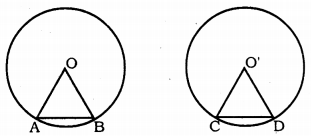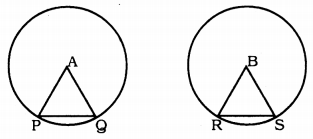# KSEEB Solutions for Class 9 Maths Chapter 12 Circles Ex 12.2

In this chapter, we provide KSEEB SSLC Class 9 Maths Chapter 12 Circles Ex 12.2 for English medium students, Which will very helpful for every student in their exams. Students can download the latest KSEEB SSLC Class 9Maths Chapter 12 Circles Ex 12.2 pdf, free KSEEB SSLC Class 9 Maths Chapter 12 Circles Ex 12.2 pdf download. Now you will get step by step solution to each question.

## Karnataka Board Class 9 Maths Chapter 12 Circles Ex 12.2

Question 1.
Recall that two circles are congruent if they have the same radii. Prove that equal chords of congruent circles subtend equal angles at their centres.
Solution:Data: Two circles having centres O and O’ and having equal radius. Chord AB = CD.
To Prove: Equal chords of congruent circles subtend equal angles at their centres.
Or ∠AOB = ∠CO’D
Proof: In ∆AOB and ∆CO’D,
OA = O’C (Data)
OB = O’D (Data)
AB = CD (Data)
∴ ∆AOB ≅ ∆CO’D
∴ ∠AOB = ∠CO’D.

Question 2.
Prove that if chords or congruent circles subtend equal angles at their centres, then the chords are equal.
Solution:Circle with centres A and B are congruent and ∠PAQ = ∠RBS
To Prove PQ = RS.
Proof: In ∆APQ and ∆BRS,
AP = BR ……… radii of congruent circles
AQ = BS are equal
∠PAQ = ∠RBS (Data)
∴ ∆PQR ≅ ∆BRS (SAS postulate)
∴ PQ = RS.

All Chapter KSEEB Solutions For Class 9 Maths

—————————————————————————–

All Subject KSEEB Solutions For Class 9

*************************************************

I think you got complete solutions for this chapter. If You have any queries regarding this chapter, please comment on the below section our subject teacher will answer you. We tried our best to give complete solutions so you got good marks in your exam.

If these solutions have helped you, you can also share kseebsolutionsfor.com to your friends.

Best of Luck!!